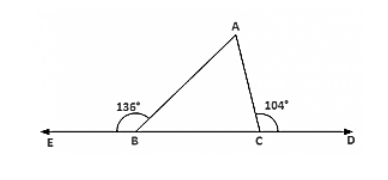# The exterior angles, obtained on producing the base of a triangle both ways are 104° and 136°. Find all the angles of the triangle.`
Question:

The exterior angles, obtained on producing the base of a triangle both ways are 104° and 136°. Find all the angles of the triangle.

Solution:

∠ACD = ∠ABC + ∠BAC [Exterior angle property]

Now ∠ABC = 180° − 136° = 44° [Linera pair]

∠ACB = 180° − 104° = 76° [Linera pair]Now, In ΔABC

∠A + ∠ABC + ∠ACB = 180° [Sum of all angles of a triangle]

⇒ ∠A + 44° + 76° = 180°

⇒ ∠A = 180° − 44° − 76°

⇒ ∠A = 60°